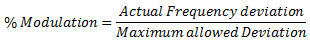# Single Tone Frequency Modulation

## Single Tone Frequency Modulation

For the single tone frequency modulation,i.e the modulating signal x(t) be a sinusoidal signal of amplitude Eand frequency fm .

Therefore,  x(t) =  Ecos (2πfmt)

The unmodulated carrier is represented by the expression :

ec  = Esin (ωct + φ)

### Instantaneous frequency of an FM wave

In FM, the frequency f of the FM wave varies in accordance with the modulating voltage .

Thus,Where Δf = kEm  and it is called as frequency deviation .

### Frequency Deviation (Δf)

Frequency deviation Δf represents the maximum departure of the instantaneous frequency fi(t) of the FM wave from the carrier frequency f.

Since Δf = kEm, the frequency deviation is proportional to the amplitude of modulating voltage (Em) and it is independent of the modulating frequency f.

### Maximum frequency of FM Wave

The maximum frequency of FM wave is given by :

fmax = f± Δf

## Mathematical Expression for FM

FM wave is a sine wave having a constant amplitude and a variable instantaneous frequency .

As the instantaneous frequency is changing continuously, the angular velocity ω of an FM wave is the function of ωand ωm .

Therefore, the FM wave is represented by,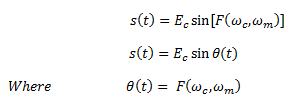As shown in fig.1, Esin Θ(t) is a rotating vector . If Eis rotating at a constant velocity ‘ω’, then we could have written that Θ(t)=ωt .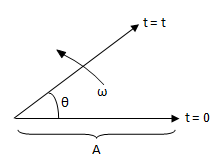Fig.1 : Frequency modulated vector

But, in FM , this velocity is not constant. In fact, it is changing continuously.

The angular velocity of FM wave is given as,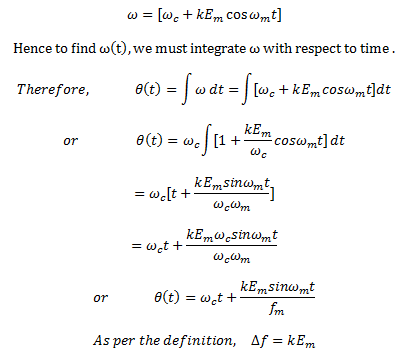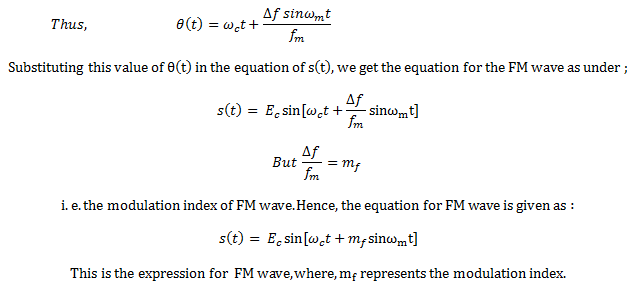### Modulation Index

The modulation index of an FM wave is defined as under :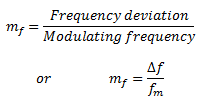The modulation index is very important in FM because it decides the bandwidth of the FM wave which we will discuss later . The modulation index also decides the number of sidebands having significant amplitudes .

In AM, the maximum value of the modulation index m is 1 . But, for FM, the modulation index can be greater than 1.

### Deviation Ratio

In FM broadcasting, the maximum value of deviation is limited to 75 kHz. The maximum modulating frequency is also limited to 15 kHz.

The modulation index corresponding to the maximum deviation and maximum modulating frequency is called as the deviation ratio .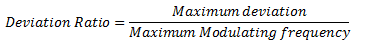### Percentage Modulation of FM Wave

The percent modulation is defined as the ratio of the actual frequency deviation produced by the modulating signal to the maximum allowable frequency deviation .

Thus,